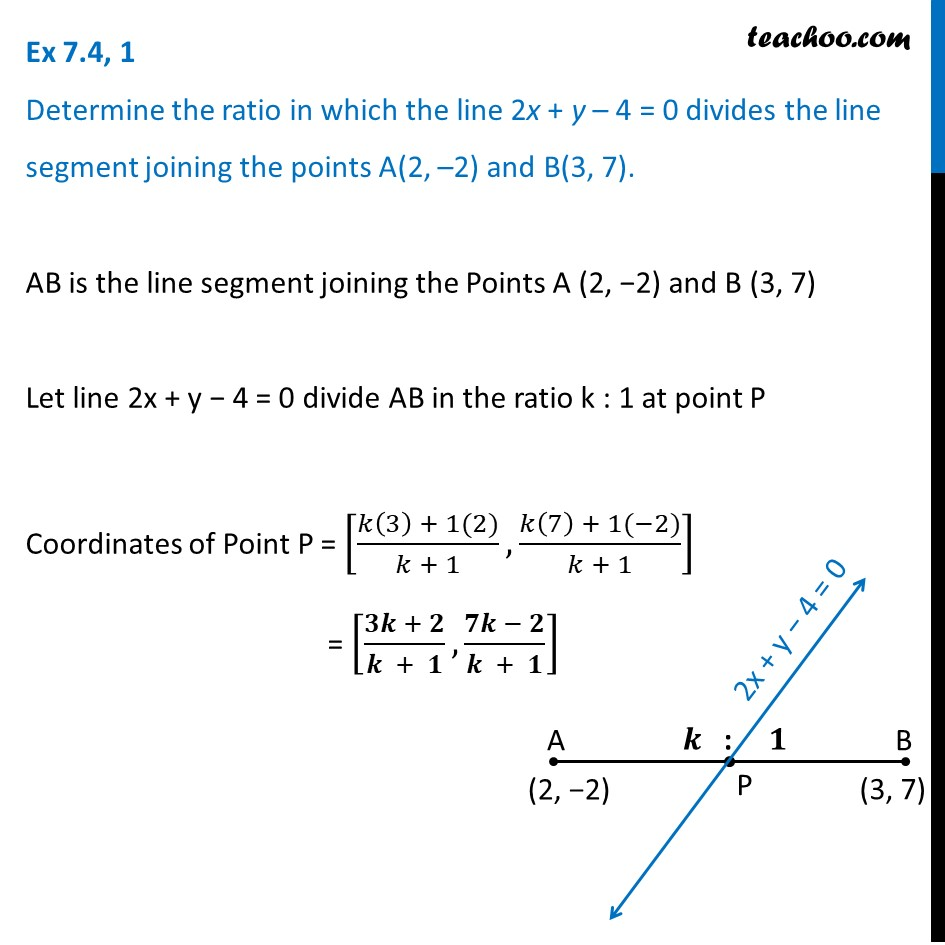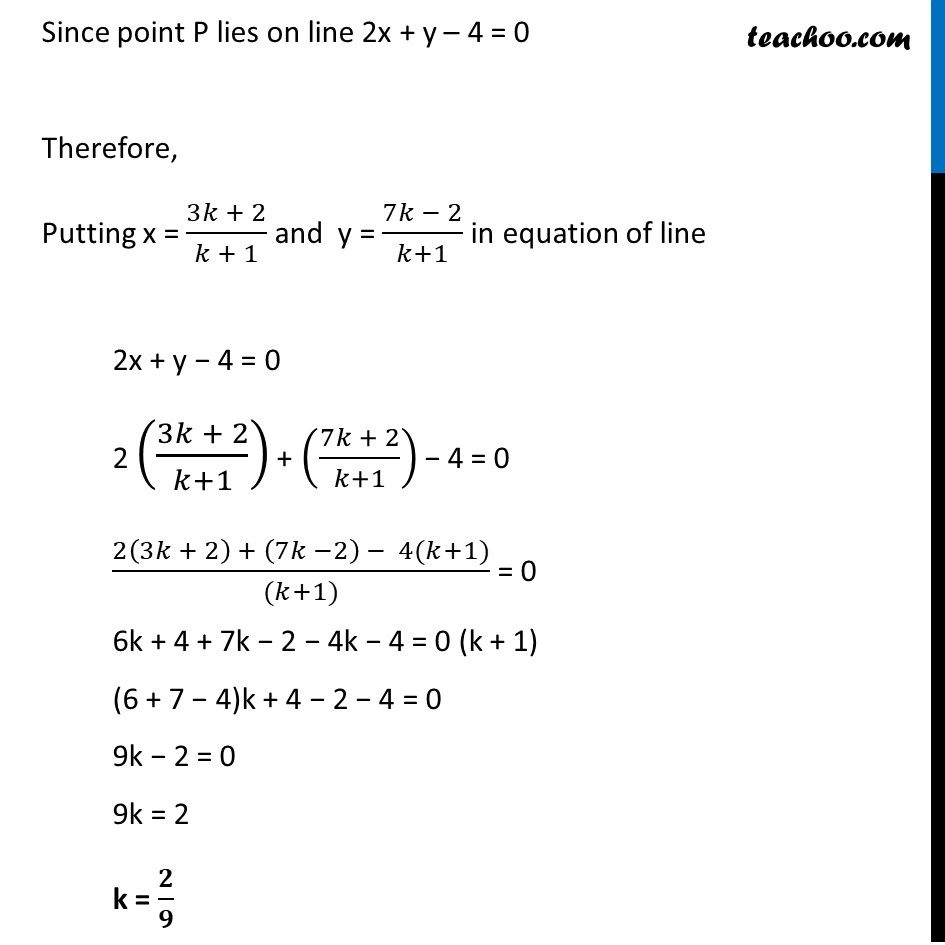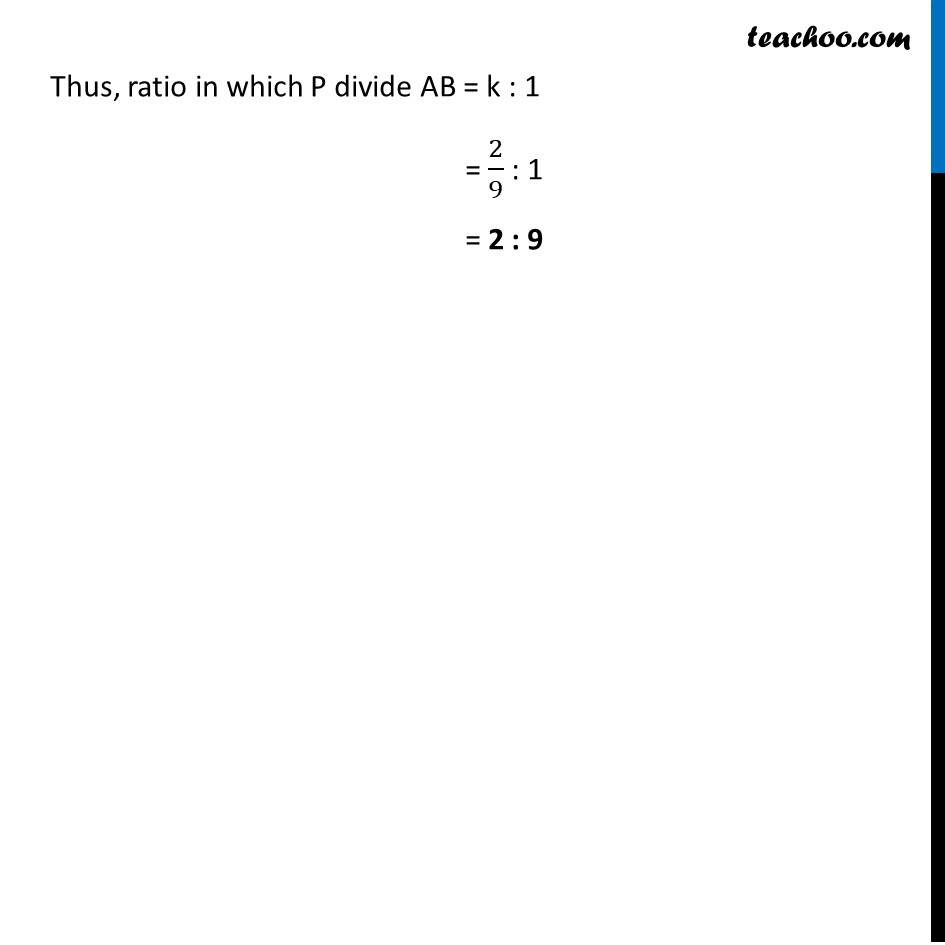Important Coordinate Geometry Questions

Chapter 7 Class 10 Coordinate Geometry
Serial order wiseLearn in your speed, with individual attention - Teachoo Maths 1-on-1 Class

### Transcript

Question 1 Determine the ratio in which the line 2x + y – 4 = 0 divides the line segment joining the points A(2, –2) and B(3, 7). AB is the line segment joining the Points A (2, −2) and B (3, 7) Let line 2x + y − 4 = 0 divide AB in the ratio k : 1 at point P Coordinates of Point P = [(𝑘(3) + 1(2))/(𝑘 + 1),(𝑘(7) + 1(−2))/(𝑘 + 1)] = [(𝟑𝒌 + 𝟐)/(𝒌 + 𝟏),(𝟕𝒌 − 𝟐)/(𝒌 + 𝟏)] Since point P lies on line 2x + y – 4 = 0 Therefore, Putting x = (3𝑘 + 2)/(𝑘 + 1) and y = (7𝑘 − 2)/(𝑘+1) in equation of line 2x + y − 4 = 0 2 ((3𝑘 + 2)/(𝑘+1)) + ((7𝑘 + 2)/(𝑘+1)) − 4 = 0 (2(3𝑘 + 2) + (7𝑘 −2) − 4(𝑘+1))/((𝑘+1)) = 0 6k + 4 + 7k − 2 − 4k − 4 = 0 (k + 1) (6 + 7 − 4)k + 4 − 2 − 4 = 0 9k − 2 = 0 9k = 2 k = 𝟐/𝟗 Thus, ratio in which P divide AB = k : 1 = 2/9 : 1 = 2 : 9